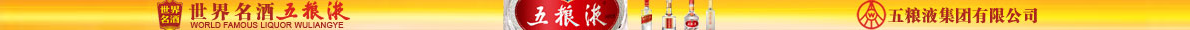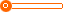# 茅台镇白酒,低价白酒,优质酱香型怀羲酒 原酒批发

60 瓶

10000 瓶

(发货期限：自买家付款之日起 天内发货)

• VIP指数:[第1年]
• 认证信息:
• 所在地区：贵州
•• 详细说明
• 规格参数
• 联系方式
r

r  r

r

r
r

r

r       贵州怀羲酒业销售有限公司，是一家以纯酱香型白酒为主打的酿造企业，酒厂坐落在美丽的国酒之乡茅台镇，此地出产的美酒，可上溯至西汉元光五年汉武帝御为贡酒起，便已名扬天下。公司主要从事酱香型白酒研发、销售的企业。拥有完整、科学的质量管理体系。本厂位于中国酒都贵州省仁怀市茅台镇，本厂年生产粮酒量在1000吨左右。 本公司销售范围较广，可长年为客户提供3到10年存酿基酒。主要经营贵州怀羲酒、赖茅酒、贵州茅台系列酒、茅台镇酱香基酒、贴牌，加工、定制专用酒等。 r

r

r   r

r

r
r
r
r
r产品实物拍摄： r

r

r
r

r

r
r
r
r
r
r
r
r
r
r
r
r
r r

r

r
r

r

r
r

r

r 酱香型白酒的生产工艺： r

r

r
r

r

r     r

r

r           茅台镇传统的酱香型白酒生产工艺严格按照节气进行，季节性很强，通常是端午采曲、重阳投料。
r
茅台镇传统生产工艺生产的酱香型白酒，其基酒生产周期长达一年，生产工艺的步骤分为清蒸下沙、混蒸糙沙二次投料；一到七个烤酒轮次，可概括为二次投料、九次蒸煮、八次发酵、七次取酒，历经春、夏、秋、冬一年时间，这也是贵州茅台酒的987生产工艺。
r
r        酱香型白酒生产工艺的第一步是清蒸下沙：采用总投料量的一半，经润粮
配料上甑蒸粮下甑泼量水摊凉洒酒尾撒曲堆积下窖封窖发酵开窖取醅为清蒸下沙工艺流程；
r
r       酱香型白酒生产工艺的第二步是混蒸糙沙：采用总投料量的另一半，经润粮
配料（加入一次清蒸下沙后的醅料）上甑蒸粮蒸酒（这次蒸出的酒不作正品，泼回酒窖重新发酵)下甑泼量水摊凉洒酒尾撒曲堆积下窖封窖发酵开窖取醅为混蒸糙沙工艺流程；
r
r      酱香型白酒生产工艺中九次蒸煮：清蒸下沙一次，混蒸糙沙一次，混蒸糙沙后的醅料
上甑蒸酒为第三次蒸煮，第三次蒸煮后的醅料为熟糟，熟糟经摊凉撒曲堆积下窖封窖发酵开窖取醅上甑蒸酒六个轮次循环过程中有六次蒸煮，共九次蒸煮。
r
r       酱香型白酒生产工艺中八次发酵：清蒸下沙一次，混蒸糙沙一次，熟糟
上甑蒸酒六个轮次循环过程中有六次封窖发酵，每加曲入窖发酵一个月，共八次发酵。
r
r       酱香型白酒生产工艺中七次取酒：混蒸糙沙上甑蒸酒后第一次取酒，熟糟
上甑蒸酒六个轮次循环后取六次酒，共七次取酒。经七次取酒后的酒糟为丢糟。
r
r
贵州茅台镇按传统工艺生产酱香型白酒的企业，都严格按照以上酱香型白酒生产工艺进行生产，这也是茅台酒的生产工艺，生产出来的各轮次酒的质量各有特点，应分质贮存，三年后进行盘勾。盘勾后再贮存一年，经微调后出厂。
r

r

r
r

r

r
r

r

r 品鉴方法： r

r

r
r

r

r 一、开封验其真 r

r

r   r

r

r 主要是打开包装后，就初步可以鉴别是酒还是水，是酱香型白酒还是浓香型白酒、清香型白酒等。 r

r

r   r

r

r 二、荡香观其色   r

r

r   r

r

r 主要看酒的颜色和粘稠度等。将酒倒入杯中，观察酒的颜色、黏稠度、挂杯效果等。酱香型中的陈年好酒，颜色会发黄，酒象胶水一样粘稠，倒在杯中，酒会沿着酒杯壁慢慢往上蔓延，达到一定得高度；而一般的酱香型白酒虽然颜色也会发黄，但是会象水一样没有黏稠度，在酒杯壁上蔓延的高度很小。造成这种情况的主要原因是陈年老酒的酒精分子聚合了，形成了大分子的酒精，分子间的作用力大，整体的表面张力大，而时间短的酱香型. 白酒的酒精分子没有聚合，还是小分子，分子间的作用力小，整体的表面张力小。 r

r

r   r

r

r 三、咂香品其味 r

r

r
r

r

r
r

r

r 公司资质： r

r

r
r

r

r
r

r

r
r

r

r
r

r

r
r
r
r
r
r
r

r

r
r

r

r
r

r

r 本公司面向全国诚征经销商，低门槛，高回报，只要您有创业梦想，我们期待您的加入!! r

r

r
r

r

r
r

r"};

查看更多同类招商加盟产品
上一组 下一组

## 您可以通过以下类目找到类似信息：

免责声明：以上所展示的信息由会员自行提供，内容的真实性、准确性和合法性由发布会员负责。中国酒客网对此不承担任何责任。

友情提醒：为规避购买风险，建议您在购买相关产品前务必确认供应商资质及产品质量！